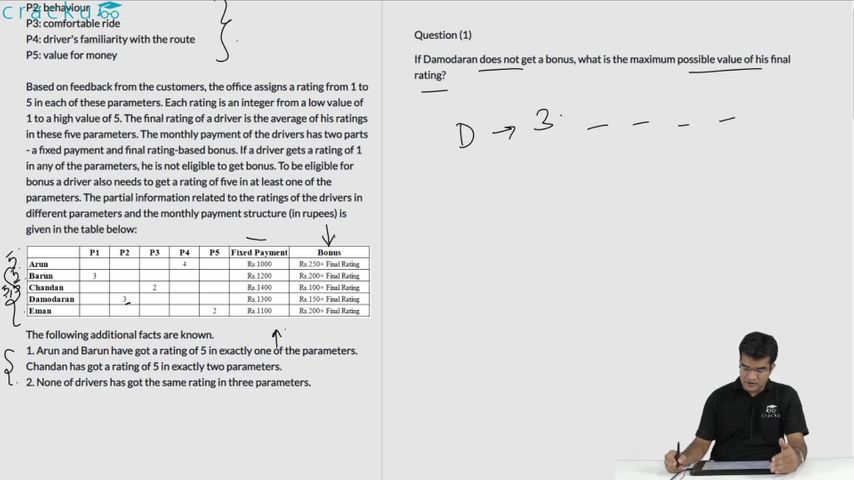### CAT 2020 Question Paper (Slot 1) Question 39

Instructions

The local office of the APP-CAB company evaluates the performance of five cab drivers, Arun, Barun, Chandan, Damodaran, and Eman for their monthly payment based on ratings in five different parameters (P1 to P5) as given below:
P1: timely arrival
P2: behaviour
P3: comfortable ride
P4: driver's familiarity with the route
P5: value for money

Based on feedback from the customers, the office assigns a rating from 1 to 5 in each of these parameters. Each rating is an integer from a low value of 1 to a high value of 5. The final rating of a driver is the average of his ratings in these five parameters. The monthly payment of the drivers has two parts - a fixed payment and final rating-based bonus. If a driver gets a rating of 1 in any of the parameters, he is not eligible to get bonus. To be eligible for bonus a driver also needs to get a rating of five in at least one of the parameters. The partial information related to the ratings of the drivers in different parameters and the monthly payment structure (in rupees) is given in the table below:

The following additional facts are known.
1. Arun and Barun have got a rating of 5 in exactly one of the parameters. Chandan has got a rating of 5 in exactly two parameters.
2. None of drivers has got the same rating in three parameters.

Question 39

# If all five drivers get bonus, what is the minimum possible value of the monthly payment (in rupees) that a driver gets?

Solution

Our objective here is to minimize the final ratings in order to find the minimum value of the monthly payment. We cannot have a rating of 1 in any of the parameters since all the drives got the bonus and we need to have at least one parameter with a rating of 5. With this understanding, we obtain the following:

Arun:

Final rating = (5+4+2+2+3)/5 = 16/5 = 3.2; Fixed payment = Rs.1000

Variable payment = 3.2 * Rs. 250 = Rs.800 ; Total = Rs.(1000+800) = Rs. 1800

Barun:

Final rating = (5+3+2+2+3)/5 = 15/5 = 3; Fixed payment = Rs.1200

Variable payment = 3 * Rs. 200 = Rs.600 ; Total = Rs.(1200+600) = Rs. 1800

Chandan: {rating of 5 in exactly two parameters based on condition 1}

Final rating = (5+5+2+2+3)/5 = 17/5 = 3.4; Fixed payment = Rs.1400

Variable payment = 3.4 * Rs. 100 = Rs.340 ; Total = Rs.(1400+340) = Rs. 1740

Damodaran:

Final rating = (5+3+2+2+3)/5 = 15/5 = 3; Fixed payment = Rs.1300

Variable payment = 3 * Rs. 150 = Rs.450 ; Total = Rs.(1300+450) = Rs. 1750

Eman:

Final rating = (5+3+2+2+3)/5 = 15/5 = 3; Fixed payment = Rs.1100

Variable payment = 3 * Rs. 200 = Rs.600 ; Total = Rs.(1100+600) = Rs. 1700

Hence, we observe that the minimum value of the monthly payment is Rs. 1700. Option D is the correct answer.

### View Video Solution